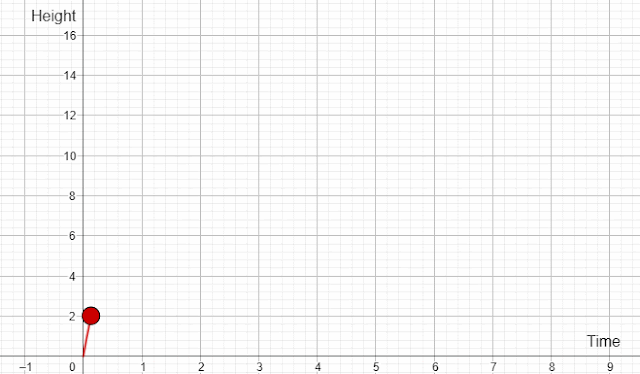### Modelling with Differentiation for A Level Maths and PhysicsMotion under gravity - modelling with differentiation

The motion of an object under gravity can easily be modelled by differentiation.

Suppose the motion of a stone is given by the formula, y = 16t - 4t2, where y and t are displacement and time respectively.

y = 16t - 4t2

dy/dt = 16 - 8t

When the stone reaches the highest point, dy/dt = 0

16 - 8t = 0

8t = 16

t = 2s

Maximum height reached by the stone = 16x2 - 4x22 = 16m

d2y/dx2 = -8 < 0

Therefore, when t = 2s, the height reaches the maximum.

In order to play with the simulation interactively, please use the following and play with the slider: